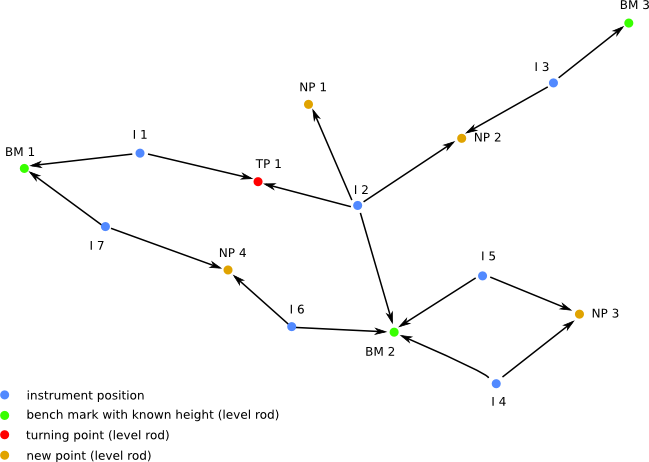# Height calculation from levelling

 Branched levelling Example 4 describes a measurement with closed loops. The path from the defined benchmark BM 1 to the respective measuring point can now take 2 paths and with I4, I5 and NP 3 even 4 paths. Accordingly, for each point more than one results are displayed. The script calculates the average of the results and the measuring/reading error by calculating the difference between the highest and lowest value (span width). There is no limit to the number of paths that can be generated by combining closed measurement paths. However, it does not make sense to define measurement paths that result in a large number of paths. Increasing the number of turning points may result in adding up of reading errors. This should be taken into account when interpreting the values for each path.Example 4